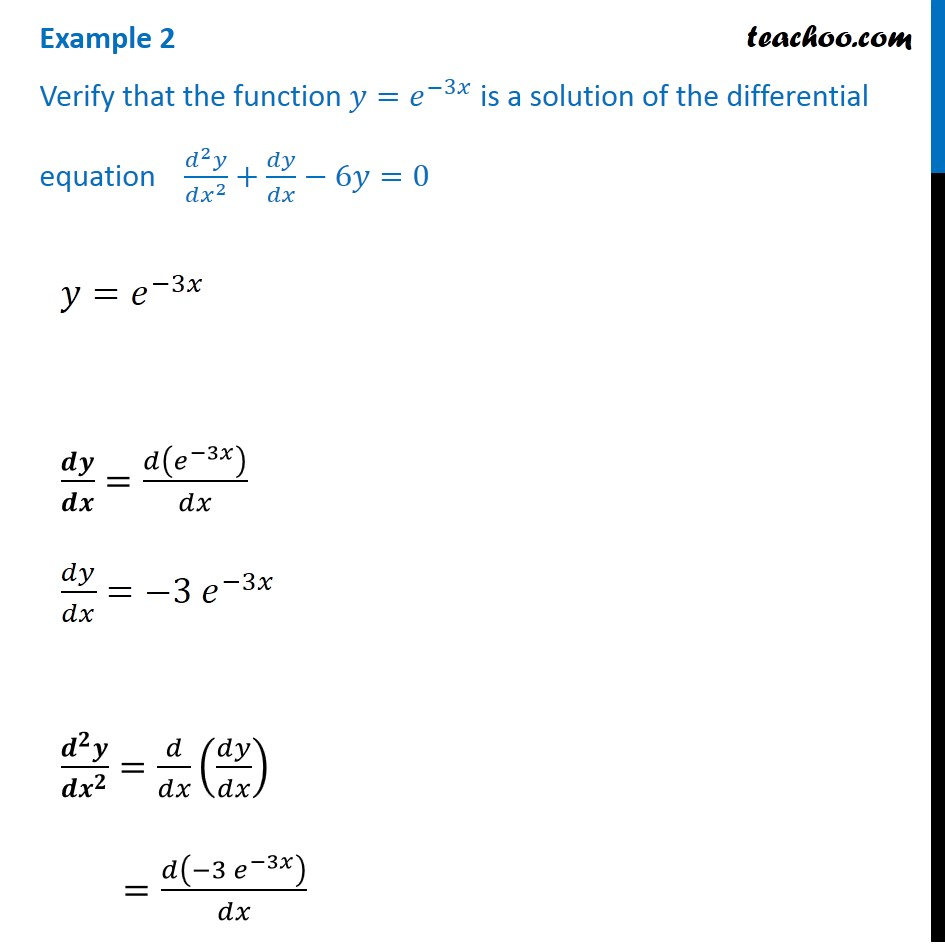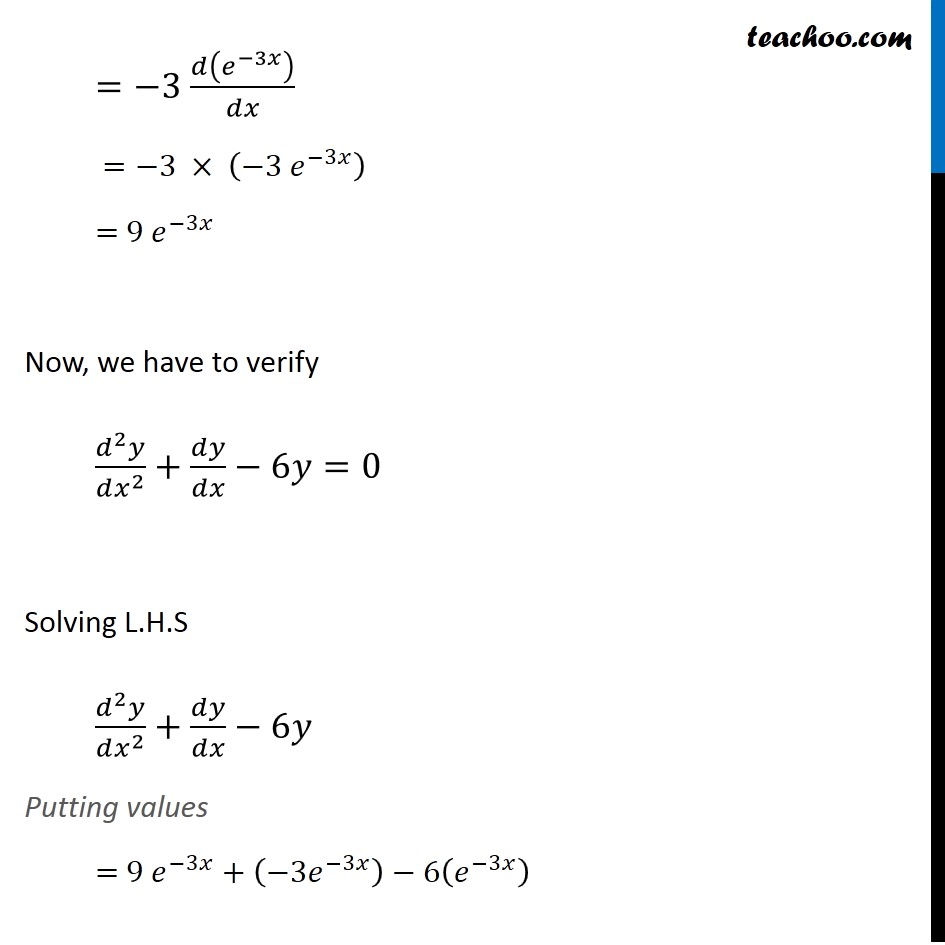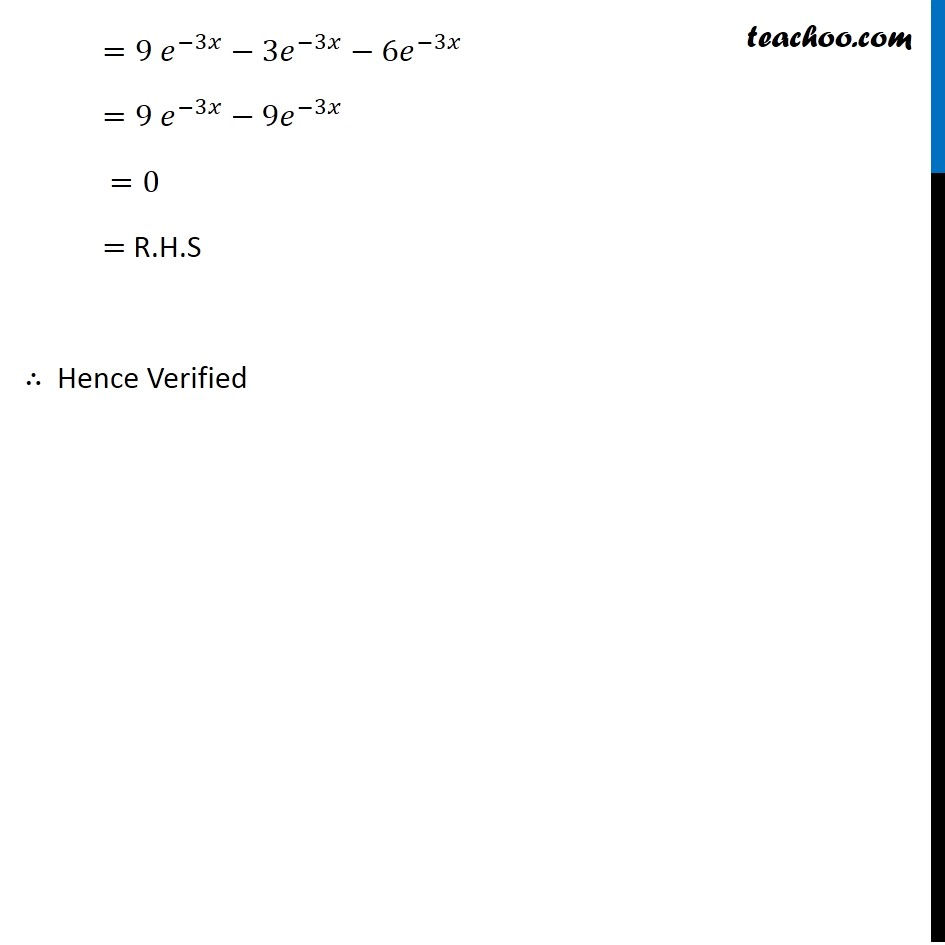Examples

Chapter 9 Class 12 Differential Equations
Serial order wiseLearn in your speed, with individual attention - Teachoo Maths 1-on-1 Class

### Transcript

Example 2 Verify that the function 𝑦=𝑒^(−3𝑥) is a solution of the differential equation (𝑑^2 𝑦)/(𝑑𝑥^2 )+𝑑𝑦/𝑑𝑥−6𝑦=0 𝑦=𝑒^(−3𝑥) 𝒅𝒚/𝒅𝒙=𝑑(𝑒^(−3𝑥) )/𝑑𝑥 𝑑𝑦/𝑑𝑥=〖−3 𝑒〗^(−3𝑥) (𝒅^𝟐 𝒚)/(𝒅𝒙^𝟐 )=𝑑/𝑑𝑥 (𝑑𝑦/𝑑𝑥) =𝑑(〖−3 𝑒〗^(−3𝑥) )/𝑑𝑥 =−3 𝑑(𝑒^(−3𝑥) )/𝑑𝑥 =−3 × (〖−3 𝑒〗^(−3𝑥) ) = 〖9 𝑒〗^(−3𝑥) Now, we have to verify (𝑑^2 𝑦)/(𝑑𝑥^2 )+𝑑𝑦/𝑑𝑥−6𝑦=0 Solving L.H.S (𝑑^2 𝑦)/(𝑑𝑥^2 )+𝑑𝑦/𝑑𝑥−6𝑦 Putting values = 〖9 𝑒〗^(−3𝑥)+(−3𝑒^(−3𝑥) )−6(𝑒^(−3𝑥) ) =〖9 𝑒〗^(−3𝑥)−3𝑒^(−3𝑥)−6𝑒^(−3𝑥) =〖9 𝑒〗^(−3𝑥)−9𝑒^(−3𝑥) =0 = R.H.S ∴ Hence Verified